+64 7 350 3209 +61 2 8011 3645 info@interpine.co.nz
Select Page

Practitioners Guide to the Methodology

Introduction

Concerned with improving the recovery of merchantable volume across a harvest cutover, a harvest supervisor or forest owner will often need to quantify how much is left post-harvest. The time and effort spent doing so must be put in the context of the value of the potential residue volume remaining, and hence the development of the technique of line intercept sampling (LIS).

The use of LIS for cutover residue assessment is in essence simple and well-proven, however many questions arise in its practical use. The aim of this report is to provide a quick reference to a number of practical aspects of the line intersect method for cutover residue assessment for harvest supervisors wishing to understand and implement this technique in different conditions. This is not planned as a conventional review of literature, nor does it introduce any new theory. Instead, it is intended to fill the gap between theory and procedure handbook and to promote the best possible understanding of the method, combined with a brief simulation and worked example.

Line Intercept Sampling

Since its original description for merchantable cutover residue assessment in Kaingaroa Forest by Warren and Olsen in 1964, the line intersect method (LIS) has been extensively used for measuring the quantity of wood lying on or near the ground. A number of people over the years continued to develop the technique (Bailey 1970, Wagner and Wilson 1976, Hall 1986, Bell et al 1996), proof its mathematical basis in depth and extend its application (De Vries 1973), review the practical aspects of LIS to overcome bias and improve precision (Wagner, 1982), and carry out a series of simulation studies (Pickford and Hazard 1978, Bell et al. 1996).

Understanding the Nature of the Line Intersect Method

The line intercept is best pictured as a strip sample of infinitesimal width (Figure 1). The data collected are diameters of wood pieces at their points of intersection with a sample line. The sample line is really a vertical plane, and the tally in effect collects a series of circular cross-sectional areas from the intersected wood pieces (Wagner, 1982).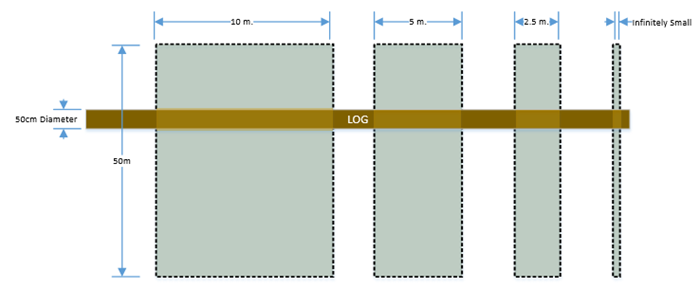Transect A Transect B Transect C Transect D (LIS) Transect Width (m) 10 5 2.5 Infinitely Small Transect Length (m) 50 50 50 50 Area (m2) 500 250 125 Infinitely Small Piece Diameter (cm) 50 50 50 50 Plot Volume (m3) 2.0 1.0 0.5 Infinitely Small Volume Per Area (m3/ha) 39 39 39 39*1

Note: *1 probability theory factor removed for this example as piece is a cylinder and crosses at right angles

Figure 1 – Picturing a strip sample of infinitesimal width

Of course the log shown in Figure 1 cross at right angles for each transect, which is often not the case. Therefore the actual cross sectional areas are really ellipses of various shapes, but a factor derived from probability theory (π/2) allows the cross sectional area to be summed as circles.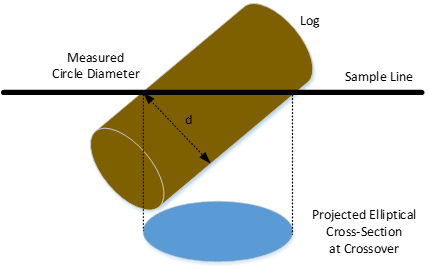Figure 2 – The elliptical cross-section at the intersection of a sample line. Addition of the probability theory factor means only ‘d’ needs to be measured above.

Figure 1, also represents a log with no taper which is often not the case. Because LIS simply collects an unbiased sample of cross sections from the wood pieces lying on the ground, Pickford and Hazard 1978 found by way of a range of simulation studies that LIS was unaffected by any kind of variation in diameter throughout the length of the pieces.

The basic equation is then derived (Wagner 1968):

Equation 1: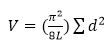Where: V= volume per unit area (m3/ha), d= piece diameter at intersection (cm), L= length of the sample line (m), π2/8= product of π/2 (probability theory factor mentioned above) and π/4 (factor to convert d2 to circular area).

Assumptions for use of LIS

LIS as described in Equation 1 embodies several assumptions which are important to consider when implementing or considering any changes to procedure manuals.

Assumption Practical Consideration
Ground Slope Quoting the resulting volume for residue assessment is by way of horizontal map area. Therefore it is important to correct all transects either by way of a slope correction factor after data collection or by increasing the transect line length during data collection. This is usually done for each transect segment. The latter is the recommended norm during cutover residue assessment. It is worthy of comment that fire fuel loading is often quoted as actual ground area rather than horizontal map area, therefore slope correction is not done (Wagner, 1982).

Equation 2: Slope Distance = (distance)/cos(slope*π/180) Where: slope= degrees

Pieces Occur Horizontally. The basic equation assumes all pieces are horizontal (or on the ground plane when considered with slope adjustment). If a piece is tilted, its probability of being intersected by the sample line is obviously lessened. If many pieces are tilted there will be fewer intersections for a given wood volume. A correction factor can be calculated 1/COS*h where h is the angle of tilt from horizontal (Wagner, 1982). This error is very low at low angles, being 0.4% at 5 degrees (h) and <10% at 25 degrees (h). Whether it is worth correcting for this error is a matter of judgement for an individual survey design. If only the larger merchantable material (commonly 3-3.7m in length and 10cm SED) is being assessed this will often be dealt with in a practical manner in the field by estimating the lay of the piece in the ground plane (Figure 3a). The most error arises measuring slash (branch and top material) especially where the smaller pieces are attached to larger ones (Figure 3b).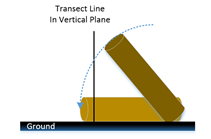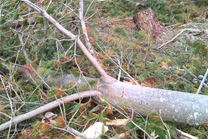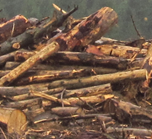Figure 3 (a) simple consideration of pieces occurring off horizontal (b) example of difficulty in assessing branch material for tilt when attached to larger pieces, but across the cutover most merchantable size volume on cutover is already be lying on the ground plane. (c) difficulty of using LIS across a slash pile due to this assumption with “most” pieces unable to be easily assessed for tilt from ground plane.

Piece Orientation is Random The basic equation assumes all pieces are randomly orientated, with all angles equally represented. However orientation bias, in which pieces are lined up in more than one direction than another, can result from windthrow residue or different styles of logging (cable, cut to length at stump, skidder). Practical difficulty is that it may be obvious or it may not be. It has been shown this can contribute to the largest potential bias and precision in the use of LIS. However since orientation bias can easily be neutralised through transect sample layout, it makes sense to always design a sample layout to insure against it. The most common method currently used in New Zealand for cutover residue assessment is a right angle transect where a single 50m transect is divided into two 25m transects at right angles (Figure 4a). However it was shown by Wagner (1968) and again reproofed by Bell et al (1996) that the best unbiased method was an equilateral triangle (Figure 4b). However as shown again in 2002 by Linnell Nemec and Davis, the difference in time associated with layout favours the use of right angled transect being some 50% faster to install compared with the equilateral triangle, and allowing the extra transect line distance to be spread out more across the cutover area (same total transect sample length split across more transect plots). Therefore the right angled transect has remained popular and widely implemented, and is equally recommended here. The length of each line transect plot is discussed in section 1.2.3 regarding sample size, but of interest 50m was derived by Warren and Olsen in the 1964 procedure of using transects of ¼ chain in length which is ~50m. Many have experimented with this length but it remains in common use today.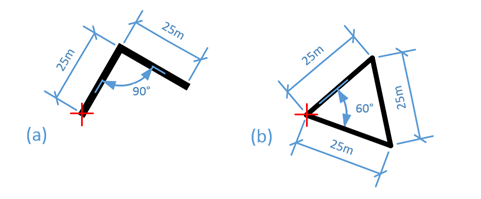Figure 4 (a) 25m Right Angled Transect (b) 25m Equilateral Triangle

With the shape of individual transects selected it is important to consider the wider layout of these transects to reduce orientation bias. In 1996 a method of creating baselines and a continuous transect of linked right angled transects was also proposed by Hall. This seems to have not been adopted into practice due to the need to adjust baselines in field based on extracted haul directions and difficulty in ensuring the total transect length is optimised across the cutover area (Figure 5c). Therefore a systematic grid-point system is favoured for locating LIS start points and these being easily spaced throughout the cutover to meet the total transect line length sample requirements. Some procedures implement a unidirectional layout of transect (Figure 5a), primarily because of the distinct time advantage over a fully random orientated layout (minimising walk distance between ends of transect and next start point). Howard and Ward (1972) concluded this was suitable comprise for residue assessment on flat to rolling terrain where tractor logging is practiced because the piece layout is generally random. However a random orientation pattern is recommended by most research to date where any bias in piece orientation is suspected (Figure 5b). This is important as when we assume the probability theory factor (π/2), piece orientation must be random either by the nature of the pieces or the random orientation of the transects.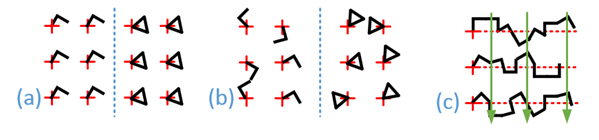Figure 5 (a) systemic unidirectional transect layout (b) random orientation transect layout (c) continuous transects on baselines perpendicular to haul extraction direction (green arrows)

Pieces are Round A non-circular piece cross section could introduce error if only measured in one dimension with a calliper. This is because πr2 is used to calculate cross sectional area (functional form shown in Equation 1 is d2π/4). The simplest way to handle this is take two measurements and average providing a representative diameter as shown in Figure 6. This is recommended for all pieces >25cm in diameter, or obviously out of round (Wagner, 1982). Of interest formulae are available for when pieces are of different shapes (Wagner, 1982). DeVries (1973) also enhanced the versatility of Equation 1 by allowing the collection of direct attributes such as piece volume (through collection of LED,SED, and Length and traditional log volume equations), yet still aggregating these to a per unit area. This however does reduce the efficiency of LIS with more data needed for each intersection point and could add potential shape bias (crutch zones, mid piece diameter reductions, chamfer zones do not often adhere to standard paraboloid or cylinder volume formulas). If volume only is required Equation 1 is still the preferred method for cutover residue assessment.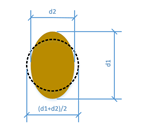Figure 6 representative cross sectional area from two calliper diameter measurements averaged.

Intercepts are Independent All intercepts with the transect line are independent. If a piece intercepts the line more than once this is counted as many times as it intercepts as shown in Figure 7. In practice an example of this is if at one intercept a piece is considered merchantable volume yet when the same piece is intercepted again it is considered non merchantable, and only merchantable intercept is measured. If both were merchantable at cross-over both are measured.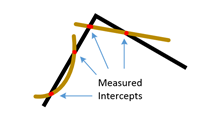Figure 7 All intercepts are considered independently of pieces, in this case there are 4 intercepts considered for measurement.

Definition of Volume The LIS methodology provides a method for calculation of harvest cutover assessment volume of round pieces (Equation 1). This volume in itself is not defined, nor is the LIS methodology linked to any assumptions of this definition. Therefore it is important for implementation to define the target volume which is desired to be assessed across a harvest cutover (e.g. at least merchantable pulp >3m in log length and 10cm SED with no rot, shatter, or excessive sweep >SED/1). Once this volume classification is defined, a LIS transect will only focus on intercepts that meet this criteria, ignoring any volume encountered. A common set of volume types surveyed in New Zealand at time of writing is shown in the Table below.

Table 1 Common Merchantable Volume Definitions in New Zealand

 Type SED LED Length Sweep Comment Pulp >=10cm n.a >=3.7 <=SED/1 No rot, insect damage or internal shatter Pulp >=10cm n.a >=3.0 <=SED/1 No rot, insect damage or internal shatter Binwood >=10cm n.a >=0.8 <=var. <=SED/1 No rot, insect damage or internal shatter Extractable Size >=10cm var. var. <=SED/1 Must be >=0.1m3 with no rot, insect damage or internal shatter

Sample Intensity

The precision of the result and hence resulting sample size, as in all sampling procedures depends on the size of the sample and the variability of the residue material assessed.

Before making some practical guidelines the following key principals identified by Wagner (1982) are worthy of taking time to consider:

1. The level of precision depends primarily on the total size of the sample (total transect line length, not the number of smaller transect sections). Therefore it is not the number of transect sections/hectare that is important but the sum of those sections to the total length of sample e.g. 34 transects*50m=1700m.
2. Theoretically, the size of the cutover area sampled is irrelevant, it is the variability of the material being sampled that counts.
3. It follows that for a given total line length the number of sections is within limits immaterial. For example; 20 sections of 50m should provide the same standard error as 10 sections of 100m each.
4. Transect sections may be either physically separated or parts of a longer continuous line.
5. Precision is also related to concentration, that is to the number of intersections per unit length of transect line.
6. Unnecessary precision is costly, to double the precision (half the standard error), would require for times the total transect length.

These principles require some practical comments: It seems unrealistic to spread a scatter of transect lines across a too larger area (point 2). It also makes sense to cover the entire cutover area with several shorter transects than few larger transects (point 3). This helps by taking advantage that transects can be separated (point 4) and provides better statistical understanding of the concentration of pieces across the cutover (point 5). It is recommended that this is done in at least 10, but preferably 20 transect sections to gain stability in the precision (point 3 and 5). It is important that these transect sections be independent from each other for the principals of random sampling to apply, therefore they must not be too close nor too short to provide this (hence joining of 2 or 3 sections for the right angled transect or the equilateral triangle transect and distributing these on a systematic grid). Precision of 20-30% probable limit of error (PLE (95%CI/mean)) will suit most cutover residue assessment applications, but for some it might be that 40% PLE would be acceptable (point 6). For example 40% PLE on a cutover with 20m3/ha merchantable volume is ±8m3/ha. If the tolerance is 5m3/ha you can still with great confidence conclude the cutover exceeds tolerance. If a royalty payment is due a higher precision might be required, but if this was just a key performance indicator (KPI) for the harvesting operator’s contract then this would be likely be fine.

Table 2 which has been adopted by Warren and Olsen in 1964 after a range of pilot studies still provides practical guide on total transect length by variability of residue material to be assessed. For example this indicates that for a 20-30% PLE that at least 20 to 32 line transect segments of 50m right angles (1000-1600m) will be suitable depending on the expectation of residue material onsite (or 13 to 21 equilateral triangles). Therefore many practitioners have implemented a sampling design of at least 1 day’s assessment across cutover area of any size. Then depending on the confidence required and the results further sampling can be completed.

Table 3 outlines a workflow example for initial setting of a sample size.

Table 2 Total transect length for varying residue volumes (adapted from Warren and Olsen 1964)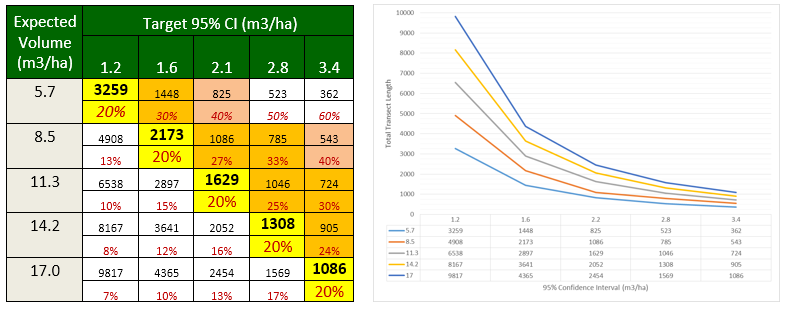*Expected PLE shown in Red, PLE of 20% shown in yellow, PLE range to 30% in orange and 40% in pink

Table 3 Practical Guideline Steps for Sample Size Definition

 Steps Example Workings to Provide an Indicative Sample Size Define expectation of residue volume 10-12m3/ha Define expectation of precision Expectation of residue merchantable <=5m3/ha onsite, 30% PLE would likely be the outer limits to provide confidence that the contractor has exceeded 5m3/ha Calculate total length of transect Total transect length 724m (from table 2) Decide on transect plot subsample type (right angled transect or equilateral triangles) Right angled transects in 50m segments. Divide the total transect length into a minimum of 10 to 20 transect sections. 15 plots Plot Layout Typically systematic random sampling grid is used, but consider using Quazi-random plot layout to allow for increased sampling if required.

Information about piece lengths, end diameters, defects can be determined by measuring additional attributes on all pieces or subsampling a portion of the transect line. This is covered in detail by Wagner (1982). One that will likely be of interest to harvest supervisors wanting to more understanding on the type of volume, is piece length distribution per unit area. This will require additional information to be collected and therefore may reduce the cost-effectiveness of the main sample. If there is a demand for this procedures could be expanded to include additional measurements or classification.

References

Bell, G. et al., 1996. Accuracy of the line intersect method of post-logging sampling under orientation bias. Forest Ecology and Management, 84(1-3), pp.23–28.

Hall, P., 1998. Logging Residue at Landings, (May), pp.1996–1998.

Howard. J, Ward. F 1972 Measurement of Logging Residue – Alterative applications of the Line Intersect Method. USDA Forest Service Research Note PNW-183

Wagner, C.E. Van, 1982. Practical aspects of the line intersect method.